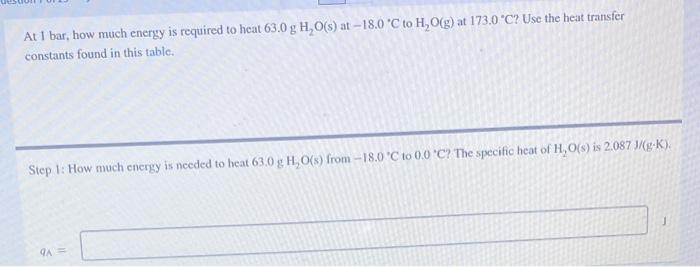Home / Expert Answers / Chemistry / at-i-bar-how-much-energy-is-required-to-heat-63-0-mathrm-g-mathrm-h-2-mathrm-o-mathrm-pa555

# (Solved): At I bar, how much energy is required to heat $$63.0 \mathrm{~g} \mathrm{H}_{2} \mathrm{O}(\mathrm ...At I bar, how much energy is required to heat \( 63.0 \mathrm{~g} \mathrm{H}_{2} \mathrm{O}(\mathrm{s})$$ at $$-18.0^{\circ} \mathrm{C}$$ to $$\mathrm{H}_{2} \mathrm{O}(\mathrm{g})$$ at $$173.0{ }^{\circ} \mathrm{C}$$ ? Use the heat transfer constants found in this table. Step 1: How much energy is needed to heat $$63.0 \mathrm{~g} \mathrm{H}_{2} \mathrm{O}(\mathrm{s})$$ from $$-18.0^{\circ} \mathrm{C}$$ to $$0.00^{\circ} \mathrm{C}$$ ? The specific heat of $$\mathrm{H}_{2} \mathrm{O}(\mathrm{s})$$ is $$2.087 \mathrm{~J} /(\mathrm{g}-\mathrm{K})$$. a)

We have an Answer from Expert